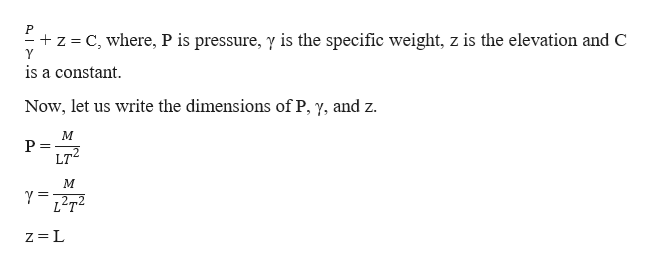# Fluid Mechanicsa) The hydrostatic equation is: (p/gamma)+z=C, where p is pressure, gamma is specific weight, and C is a constant. Show that this equation is dimensionally homogeneous.b) Would a check for dimensionless homogenity have saved the Mars Climate Orbiter? Why or why not?

Question
40 views

Fluid Mechanics

a) The hydrostatic equation is: (p/gamma)+z=C, where p is pressure, gamma is specific weight, and C is a constant. Show that this equation is dimensionally homogeneous.

b) Would a check for dimensionless homogenity have saved the Mars Climate Orbiter? Why or why not?

check_circle

Step 1

The hydrostatic equation is given, and it is to be shown that it is dimensionally homogeneous. This can be done as,help_outlineImage TranscriptioncloseP z C, where, P is pressure, y is the specific weight, z is the elevation and C Y is a constant Now, let us write the dimensions of P, y, and z M P LT M Y12T z L fullscreen
Step 2

Substituting all the respective dimensions in the equation, we get,

Step 3

Now since on the LHS of the equation, we have a dimension of length for both  and Z. Therefore, the c...

### Want to see the full answer?

See Solution

#### Want to see this answer and more?

Solutions are written by subject experts who are available 24/7. Questions are typically answered within 1 hour.*

See Solution
*Response times may vary by subject and question.
Tagged in

### Fluid Mechanics